• 半角公式 半角公式（Half angle formula）是利用某个角（如∠A）的正弦值、余弦值、正切值，及其他三角函数值，来求其半角的正弦值，余弦值，正切值，及其他三角函数值的公式。 公式 常用的半角公式包括以下三个...
半角公式

半角公式（Half angle formula）是利用某个角（如∠A）的正弦值、余弦值、正切值，及其他三角函数值，来求其半角的正弦值，余弦值，正切值，及其他三角函数值的公式。

公式

常用的半角公式包括以下三个：

半角正弦公式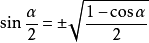半角余弦公式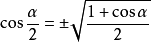半角正切公式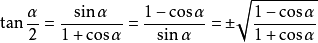以上三角函数值的正负由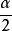所在的象限决定。

公式推导

和角公式推导：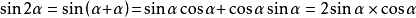半角正弦公式

由上述等式cos2α，整理得：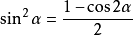将代入α，整理得：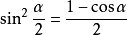开方，得半角余弦公式

由等式①整理得：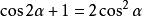将代入，整理得：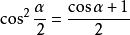开方，得半角正切公式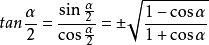展开全文三角函数
• 极限不存在有三种情况： 1.极限为无穷，很好理解，明显与极限存在定义相违。 2.左右极限不相等，例如分段函数 3.没有确定的函数值，例如lim（sinx）从0到无穷。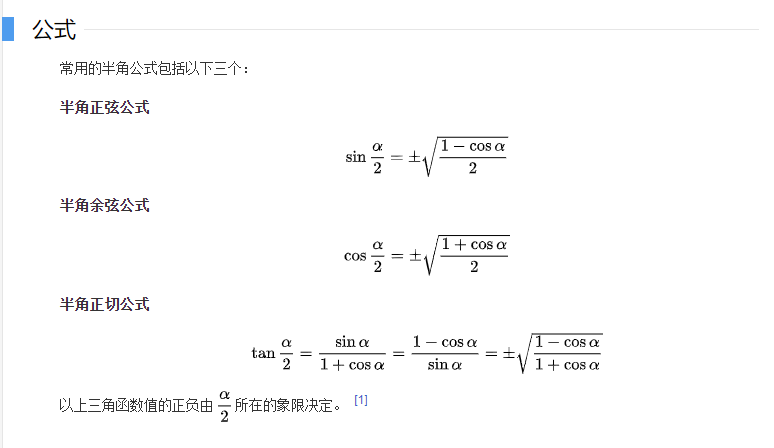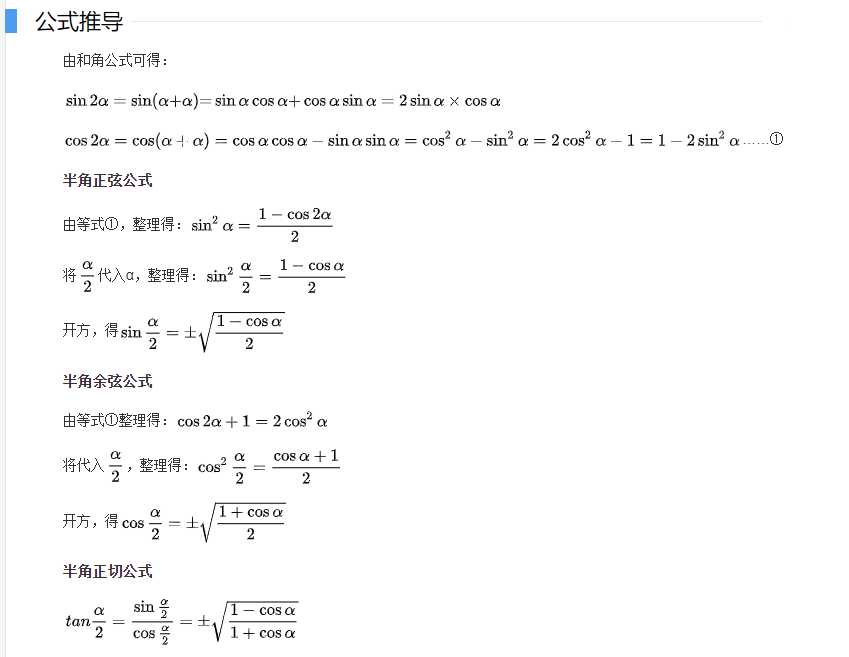极限不存在有三种情况：

1.极限为无穷，很好理解，明显与极限存在定义相违。

2.左右极限不相等，例如分段函数

3.没有确定的函数值，例如lim（sinx）从0到无穷。

展开全文• 在掌握了三角函数两角和差公式之后，我们可以根据两角和差公式，轻易地掌握三角函数倍角公式和半角公式。如果还没有掌握两角和差公式，可以先参看相关的内容，待掌握后再进行下面的环节，否则效果不佳。高中数学三角...
在掌握了三角函数两角和差公式之后，我们可以根据两角和差公式，轻易地掌握三角函数倍角公式和半角公式。如果还没有掌握两角和差公式，可以先参看相关的内容，待掌握后再进行下面的环节，否则效果不佳。高中数学三角函数公式轻松记：正弦余弦的两角和差公式“口诀”记上面是介绍的正弦和余弦的两角和差公式如何熟记，主要是应用口诀“正异同，余同异”快速掌握。高中数学三角函数公式轻松记：正切余切两角和差公式的推导与记忆上面是介绍正切和余切的两角和差公式如何推导及快速记忆之法。在这些熟练掌握后，我们就能很轻易地掌握和运用倍角公式和半角公式了。三角函数二倍角公式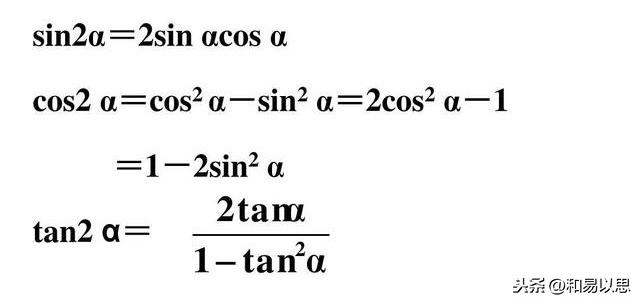三角函数二倍角公式在三角函数加法公式(即两角和差公式)中我们学习的是有两个角，其中一个用α表示，另一个用β表示。当我们现在用来记二倍角公式时，也就是一个角的2倍，而一个角的两倍就是这个角和自己相加的结果。所以我们把两角和差公式中的两个不同角变为相同的角时，两角和差公式也就成了二倍角公式。比如sin(α+β)当α=β时，sin(α+β)=sin2α=sin2β，后者不就是二倍角吗？所以只要掌握了三角函数的两角和差公式，我们把公式中的不同的两个角当作相同的角时就直接可以写出二倍角公式了。sin2α=sin(α+α)=sinαcosα+cosαsinα=2sinαcosα.同理可以写出其他形式的三角函数的二倍角公式，大家不妨自己写一下看看。这里要提示下的是余弦的二倍角公式在写出后，然后利用sinα+cosα=1这个关系式，又可以推导出两个公式。比如cos2α=cos(α＋α)根据口诀“余同异”，可以直接写出余弦的两角和的公式如下：cos2α=cos(α＋α)=cosαcosα-sinαsinα=cos²α-sin²α.①根据sin²α+cos²α=1，可以分别得出 sin²α=1-cos²α和cos²α=1-sin²α。分别代入①式就可以得出余弦的二倍角公式的另外两个常用的公式表达式了。二倍角公式上面是推理来的，如果不推理，也可以利用前面介绍的方法通过观察找特点直接写出公式。比如sin2α,这是正弦，按照“正异同”知道其公式展开式中的每一项都是相异的同组组成的，所以每一项都是sinαcosα,这样看到正弦可以直接写出此项，然后前面加个2就可以了。(想想为什么)再看cos2α,根据口诀“余同异”，可以直接写出cos²α-sin²α.三角函数半角公式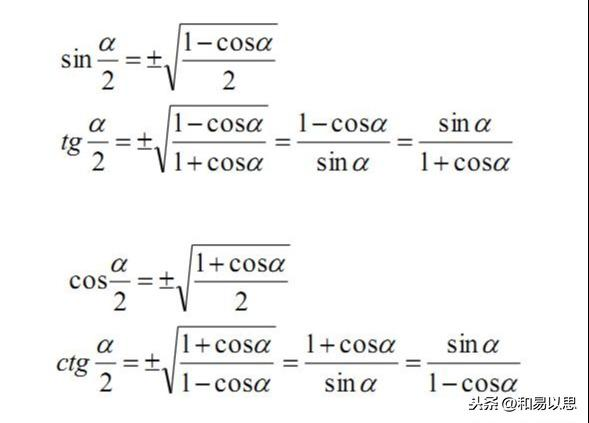三角函数半角公式这里的tg也就是tan,是正切；ctg也就是cot,是余切。不过现在教材更多采用的是tan和cot来表示正切和余切。可能大家一看公式，感觉很复杂，不好记。其实这些公式都是来自于余弦的两角和差公式。余弦的两角和差公式，当我们把两个不同的角当作相同的角看待时就变成了余弦的二倍角公式。其中一个是：cos2α=2cos²α-1 ②另一个是： cos2α=1-2sin²α ③为何采用余弦的二倍角公式而不是正弦的二倍角公式？原因就在于正弦的二倍角公式等式右面不知一个三角函数，而是同组的相异的两个三角函数。我们现在观察②式，发现等号左边的角是 2α，等号右边的角是 α，有没有发现什么特点？右面的角是左边的角的一半！③式也同样如此。也就是说等式左边的角是右边的2倍，所以是二倍角公式。从右边的角的角度来看，右边的角是左边的角的一半，岂不就是半角公式吗？当然根据公式的常用表示法，一般展开式在右边，所以我们需要把半角放在等式的左边。由此有：②式变换为 2cos²α-1 =cos2α → 2cos²α=1+cos2α → cos²α=(1+cos2α)/2,然后开方就可以得到公式的形式。(切记：开平方结果有两个，一正一负，具体选择哪个符号，还是取决半角函数的符号)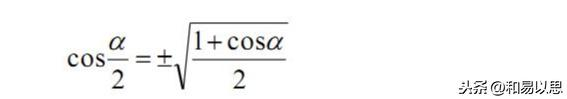余弦半角公式为了符合我们一般的习惯，我们不用α表示半角，而是用α/2表示，所以2α也对应变成了α。所以余弦的二倍角公式，因而变为：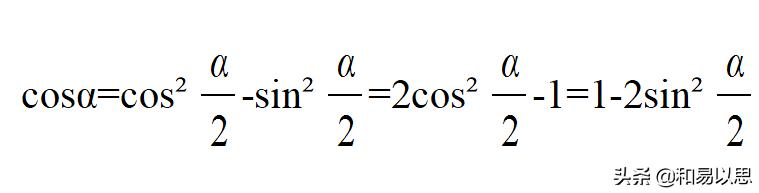根据这个式子可以得出三角函数的降幂公式，只需要再变化下即可。这个不再展开论述。这个方法也就是比较等式两边的角的关系，然后由一边的角表示另一边，这样就可以得到不同的公式，不过从根源上看还都是一个公式的变化而已。如果没有掌握这个特点，很可能会为公式繁多而忧虑，并且还很容易遗忘或记混淆。思路：余弦的两角和差公式 → 二倍角公式(令两角相等)→ 半角公式(比较等式两边角的关系) → 降幂公式。如：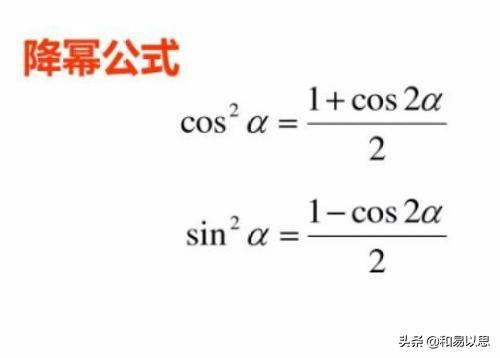三角函数降幂公式由于这里是介绍公式记忆和方法的，所以不再论述不同公式如何使用以及在具体题目中我们应该如何根据题目条件和要求确定用哪个公式。正切和余切的半角公式，也是先用正弦除以余弦公式，然后将对应的正弦和余弦的半角公式代入，最后采用分子有理化或分母有理化而分别得到两个去除根式的公式表达形式。(无论是根式中分子或分母中的1+cosα还是1-cosα,都是采用构成1-cosα的形式进行分母或分子有理化的，因为这样就是sinα,可以直接开出来了。这也是平方差公式的逆运用。)
展开全文正弦函数
• 两角和与差公式： sin⁡(α±β)=sin⁡αcos⁡β±cos⁡αsin⁡β\sin (\alpha \pm \beta)=\sin \alpha \cos \beta \pm \cos \alpha \sin \betasin(α±β)=sinαcosβ±cosαsinβ cos⁡(α±β)=cos⁡αcos⁡β∓...
两角和与差公式：
$\sin (\alpha \pm \beta)=\sin \alpha \cos \beta \pm \cos \alpha \sin \beta$
$\cos (\alpha \pm \beta)=\cos \alpha \cos \beta \mp \sin \alpha \sin \beta$
$\tan (\alpha \pm \beta)=\frac{\tan \alpha \pm \tan \beta}{1 \mp \tan \alpha \tan \beta}$
证：$\sin (\alpha+\beta)=\sin \alpha \cos \beta+\cos \alpha \sin \beta$
在笛卡尔坐标系中以原点O为圆心作单位圆，在单位圆中作以下线段如图中所示，容易看出：$\sin (\alpha+\beta)=C F ; \quad \sin \alpha=A B ; \quad \cos \alpha=OB ; \quad \sin \beta=C D ; \cos \beta=OD$
则：$S_{\Delta O C A}=\frac{1}{2} \times 1 \times C F=\frac{1}{2} \times 1 \times \sin (\alpha+\beta)$
$S_{\Delta O C E}=S_{\Delta O C B}-S_{\Delta C E B}$
$=\frac{1}{2} \times O B \times C D-S_{\Delta C E B}$
$\quad=\frac{1}{2} \times \cos \alpha \times \sin \beta-S_{\Delta C E B}$
$S_{\Delta O A E}=S_{\Delta O A D}+S_{\triangle A E D}$
$=\frac{1}{2} \times O D \times A B+S_{\triangle A E D}$
$=\frac{1}{2} \times \cos \beta \times \sin \alpha+S_{\triangle A E D}$
$S_{\Delta O C A}=\frac{1}{2} \times 1 \times \sin (\alpha+\beta)= S_{\Delta O C E}+S_{\Delta O A E}=\left(\frac{1}{2} \times \cos \alpha \times \sin \beta-S_{\Delta C E B})+\left(\frac{1}{2} \times \cos \beta \times \sin \alpha+S_{\triangle A E D}\right)\right.$
容易看出, $S_{\triangle A B D}=S_{\Delta A B C}=\frac{1}{2} \times A B \times B D$ ，而 $S_{\triangle A B E}$ 是公共三角形, $\quad \therefore S_{\triangle{AED}}=S_{\triangle{CEB}},$
可得到：$\sin (\alpha+\beta)=\sin \alpha \cos \beta+\cos \alpha \sin \beta$
对于$\sin (\alpha-\beta)=\sin \alpha \cos \beta-\cos \alpha \sin \beta$，只要将 $\sin (\alpha+\beta)$ 中的 $\beta$ 唤成 $(-\beta)$ 即可。
**二倍角公式:**由和公式，当$\alpha = \beta$，得到：
$\sin 2 \alpha=2 \sin \alpha \cos \alpha$
$\cos 2 \alpha=\cos ^{2} \alpha-\sin ^{2} \alpha$
$\tan 2 \alpha=\frac{2 \tan \alpha}{1-\tan ^{2} \alpha}$
由于$\sin^{2} \alpha+\cos ^{2} \alpha=1$，故$\cos 2 \alpha=\cos ^{2} \alpha-\sin ^{2} \alpha=1-2 \sin ^{2} \alpha=2 \cos ^{2} \alpha-1$
降幂公式： 由二倍角公式得到
$\sin ^{2} \alpha=\frac{1-\cos 2 \alpha}{2}$
$\cos ^{2} \alpha=\frac{1+\cos 2 \alpha}{2}$
半角公式：由降幂公式令$\alpha$为$\frac{\alpha}{2}$
$\sin ^{2} \frac{\alpha}{2}=\frac{1-\cos \alpha}{2} ， \cos ^{2} \frac{\alpha}{2}=\frac{1+\cos \alpha}{2}$    $\tan ^{2} \frac{\alpha}{2}=\frac{\sin ^{2} \frac{\alpha}{2}}{ \cos ^{2} \frac{\alpha}{2}}=\frac{1-\cos \alpha}{1+\cos \alpha}$
$\tan \frac{\alpha}{2}=\frac{\sin \frac{\alpha}{2}}{\cos \frac{\alpha}{2}}=\frac{\sin ^{2} \frac{\alpha}{2}}{\sin \frac{\alpha}{2} \cdot \cos \frac{\alpha}{2}}=\frac{\frac{1}{2}(1-\cos \alpha)}{\frac{1}{2} \sin \alpha}=\frac{1-\cos \alpha}{\sin \alpha}$
$\tan \frac{\alpha}{2}=\frac{\sin \frac{\alpha}{2}}{\cos \frac{\alpha}{2}}=\frac{\sin \frac{\alpha}{2} \cdot \cos \frac{\alpha}{2}}{\cos ^{2} \frac{\alpha}{2}}=\frac{\sin \alpha}{1+\cos \alpha}$
万能公式：利用二倍角证明，
$\sin 2 \alpha=2 \sin \alpha \cos \alpha=\frac{2 \sin \alpha \cos \alpha}{\cos ^{2} \alpha+\sin ^{2} \alpha}=\frac{2 \tan \alpha}{1+\tan ^{2} \alpha}$
$\cos 2 \alpha=\frac{\cos ^{2} \alpha-\sin ^{2} \alpha}{\cos ^{2} \alpha+\sin ^{2} \alpha}=\frac{1-\tan ^{2} \alpha}{1+\tan ^{2} \alpha}$
$\tan 2 \alpha=\frac{2 \tan \alpha}{1-\tan ^{2} \alpha}$
积化和差公式：由两角和与差公式，$\sin (\alpha \pm \beta)=\sin \alpha \cos \beta \pm \cos \alpha \sin \beta$，将$\sin (\alpha + \beta)$与$\sin (\alpha - \beta)$相加，得到$\sin \alpha \cos \beta=\frac{1}{2}[\sin (\alpha+\beta)+\sin (\alpha-\beta)]$，
将$\sin (\alpha + \beta)$与$\sin (\alpha - \beta)$相减，得到$\cos \alpha \sin \beta=\frac{1}{2}[\sin (\alpha+\beta)-\sin (\alpha-\beta)]$，
$\cos (\alpha \pm \beta)=\cos \alpha \cos \beta \mp \sin \alpha \sin \beta$，将$\cos (\alpha + \beta)$与$\cos (\alpha - \beta)$相加，得到$\cos \alpha \cos \beta=\frac{1}{2}[\cos (\alpha+\beta)+\cos (\alpha-\beta)]$
将$\cos(\alpha + \beta)$与$\cos(\alpha - \beta)$相减，得到$\sin \alpha \sin \beta=-\frac{1}{2}[\cos (\alpha+\beta)-\cos (\alpha-\beta)]$
和差化积公式：由积化和差公式$\sin \alpha \cos \beta=\frac{1}{2}[\sin (\alpha+\beta)+\sin (\alpha-\beta)]$，令$x=\alpha+\beta$，$y=\alpha-\beta$，代入替换$\alpha$与$\beta$， 可得到：
$\sin \alpha+\sin \beta=2 \sin \frac{\alpha+\beta}{2} \cos \frac{\alpha-\beta}{2}$
$\sin \alpha-\sin \beta=2 \cos \frac{\alpha+\beta}{2} \sin \frac{\alpha-\beta}{2}$
$\cos \alpha+\cos \beta=2 \cos \frac{\alpha+\beta}{2} \cos \frac{\alpha-\beta}{2}$
$\cos \alpha-\cos \beta=-2 \sin \frac{\alpha+\beta}{2} \sin \frac{\alpha-\beta}{2}$


展开全文• 若$\Delta ABC$满足:$\tan\dfrac{A}{2}\cdot\tan\dfrac{C}{2}=\dfrac{1}{3},b=\dfrac{4}{3}a$,则$\sin B=$______ 分析:\$\tan\dfrac{A}{2}\cdot\tan\dfrac{C}{2}=\dfrac{1-\cos A}{\sin A}\cdot\dfrac{\sin C}{1+\c...
• 主要思路:从欧拉公式推证得四条积化和差公式,得到了三角函数中加减乘除的转换基础,之后的证明就非常简单了. 1我们首先从欧拉公式推出sinx和cosx 2再推出积化和差的四个基本公式 积化和差的具体推导只是一个非技巧...
• §4 三角函数的基本关系和诱导公式 1.同角三角函数的基本公式 2.同角三角函数换算表   x x x x ...降幂公式
• Case：最近在做图纸的出版，然后就从CAD里将图纸编号直接复制到...全角转半角公式=ASC() 全角转半角公式=WIDECHAR() 使用公式将全角转换为半角之后，将公式复制粘贴为数值就可以直接将文本复制到目的表格中了。 ...excel
• 转载自http://202.113.29.3/nankaisource/mathhands/Elementary%20mathematics/0103/010306/01030601.htm 1.倍角公式
• ## 常用三角公式

千次阅读 2016-08-13 17:22:46
基本公式两角和与差公式及规律二倍角公式及规律 半角公式三倍角公式积化和差与和差化积公式万能公式皮皮blog诱导公式同角三角函数基本关系皮皮blog应注意的问题1、两角差的余弦公式是本章中其余公式的基础，应记准该...
• ## 三角函数公式

千次阅读 2018-10-17 13:43:10
目录 ...半角公式 万能公式 辅助角公式 正弦定理 余弦定理 三角和 降幂公式 基本公式   锐角三角函数 任意角三角函数 图形 直角三角形 任意角三角函数 ...
• 一、三角函数定义 二、诱导公式 三、倒数关系 四、平方关系 五、两角和与差的三角函数 六、积化和差公式 七、和差化积公式 八、倍角公式 ...九、半角公式 十、万能公式 十一、其他公式 ...三角函数
• 目录导数公式基本积分表三角函数的有理式积分初等函数两个重要极限三角函数公式和差角公式和差化积公式倍角公式半角公式正玄余弦公式莱布尼兹公式、中值定理、曲率定积分应用相关公式空间几何平面方程二次曲面多元...
• 目录：一、弧度制二、三角函数的基本定义三、同角三角函数基本关系四、三角函数的诱导公式五、三角函数的和差角公式六、倍角公式和半角公式七、积化和差与和差化积公式八、万能公式九、辅助角公式十、求导十一、反...
• 高中数学 第三章 三角恒等变换 3_2_2 半角的正弦、余弦和正切学案 新人教b版必修4 安全教育学习是提高员工安全防范意识的重要措施。“百日安全活动”开展以来，保卫部从...半角公式点睛1有了半角公式，只需知道cos...
• 考研竞赛数学|公式高等数学（篇一）（一）基础公式「初等函数」对数函数对数运算法则对数恒等式 换底公式 三角函数加法公式倍角公式半角公式和差化积公式：❝ 记忆口诀：正加正，正在前.余加余，余并肩.正减正，余在...
• 总结了高等数学微积分常用公式，适合快速查阅。包括常用： 一、基本导数公式 ...3.半角公式 4.和差化积公式 5.积化和差公式 6.万能公式 7.平方关系 8.倒数关系 9.商数关系 十五、几种常见的微分方程
• 考研竞赛数学|公式高等数学（篇一）（一）基础公式「初等函数」对数函数对数运算法则对数恒等式 换底公式 三角函数加法公式倍角公式半角公式和差化积公式：❝ 记忆口诀：正加正，正在前.余加余，余并肩.正减正，余在...
• 初等函数的图形 幂函数的图形 指数函数的图形 ...半角公式 和差化积 积化和差 诱导公式 万能公式 其它公式 其他非重点三角函数 双曲函数 公式一 ...
• 文章目录常见基本三角公式1 基本公式2 倍角公式3 半角公式4 加法公式5 和差化积公式6 积化和差公式7 万能公式8 正弦定理9 余弦定理 常见基本三角公式 1 基本公式 sin⁡2x+cos⁡2x=1:1+tan⁡2x=sec⁡2x;1+cot⁡2x=csc...
• 它是由两个角的外角平分线和另外一个角的平分线交于一点而得到的，并结合内切圆，得出与之相关的面积公式，同时会导出一些三角形的边角关系，尤其是三角形中的半角公式。如上面的三角形旁切圆图所示，圆P为三角形ABC...
• 它是由两个角的外角平分线和另外一个角的平分线交于一点而得到的，并结合内切圆，得出与之相关的面积公式，同时会导出一些三角形的边角关系，尤其是三角形中的半角公式。如上面的三角形旁切圆图所示，圆P为三角形ABC...
• 半角公式 万能公式 其他公式 反三角函数恒等式 极限相关公式 数列极限递推式 重要极限公式 常用等价无穷小 1^∞ 型 导数相关公式 导数定义 微分定义 连续，可导及可微关系 一元函数 多元函数 导数四则运算 复合函数...
• 总结了高等数学微积分常用公式，适合快速查阅，本资源为word版本，方便进一编辑排版： ...3.半角公式 4.和差化积公式 5.积化和差公式 6.万能公式 7.平方关系 8.倒数关系 9.商数关系 十五、几种常见的微分方程
• 有多少同学在数学课上看着这密密麻麻的数字公式写满了黑板，觉得十分的头疼，到底...高中必背88个数学公式有：圆的公式、椭圆公式、两角和公式、倍角公式、半角公式、和差化积、等差数列、等比数列、抛物线等公式。...
• 文章目录——写在前面的话 温馨提示：内容较长，需耐心观看目录 一、定义二、同角三角函数 三、诱导公式 四、和差角公式 五、和差化积与积化和差公式 六、倍角公式和半角公式 七、万能公式 八、辅助角公式 九、在...
• 1 基本三角公式定义： 诱导公式 基本关系 万能公式 重要基本公式  2倍角公式 半角公式 两角和差变形公式 和差化积 积化和差  ...
• 有多少同学在数学课上看着这密密麻麻的数字公式写满了黑板，觉得十分的头疼，到底应该记哪些...高中必背88个数学公式有：圆的公式、椭圆公式、两角和公式、倍角公式、半角公式、和差化积、等差数列、等比数列、抛物......